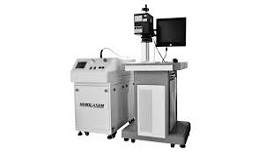## Matrix ac -- ac control

VVVF frequency conversion, vector control frequency conversion, direct torque control frequency conversion is an ac - dc - ac frequency conversion. Its common shortcoming is low input power factor, large harmonic current, large capacity of energy storage required by direct current circuit, and regenerated energy can not be fed back to the grid. That is to say, it cannot carry out four-quadrant operation. Therefore, matrix ac - ac frequency conversion arises at the historic moment. Because matrix ac - ac frequency conversion saves the intermediate dc link, saving large volume, expensive electrolytic capacitance. It can realize the power factor is l, the input current is sine and it can run in four quadrants. Although the technology is not mature, it still attracts many scholars to study it deeply. Its essence is not the indirect control current and magnetic chain equivalent, but the torque directly as the controlled quantity to achieve. The specific method is as follow.

1. Controlling stator flux to introduce stator flux observer, and realize the mode without velocity sensor.

2. Automatic identification (ID) automatically recognizes motor parameters based on accurate motor mathematical model.

3. Calculating the actual value corresponding to stator impedance, mutual inductance, magnetic saturation factor, inertia, etc. and calculating the actual torque, stator magnetic chain and rotor speed for real-time control.

4. Band - Band control is implemented to generate PWM signal by Band - Band control according to magnetic chain and torque, and controlling the inverter switch state.

Matrix ac - ac frequency has rapid torque response (<2ms), high speed accuracy (+ + 2%, no PG feedback), and high torque accuracy (<+3%). At the same time, it has high starting torque and high torque precision. Especially at low speed (including 0 speed), it can output 150% ~ 200% torque.